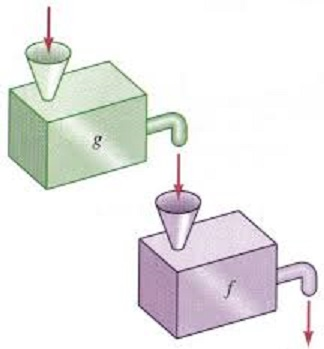# Section 2.8 - Algebraic Operations on Functions

Section Objectives

1. Compute sums, differences, products, and quotients of functions.
2. Compute a composition of functions.
3. Write a function as a composition of functions.

### Combining functions

Besides transforming individual functions, two or more functions can be combined in lots of ways to produce new functions.

The most basic combinations of functions come from the four basic arithmetic operations.

• $(f+g)(x)=f(x)+g(x)$
• $(f-g)(x)= f(x)-g(x)$
• $(fg)(x)=f(x) \cdot g(x)$
• $\displaystyle \left( \frac{f}{g} \right) (x) = \frac{f(x)}{g(x)}$, provided that $g(x) \ne 0$

#### Examples

• Given the following table of values, compute $(f+g)(2)$, $(f-g)(3)$, and $(fg)(1)$.

• Let $f(x)=x^2-7x+1$ and $g(x) = x^3$. Compute $(f/g)(2)$. What about $(f/g)(0)$?

• Sketch the graphs of $f(x)=x-3$ and $g(x)=x^2+2$. Use the graphs to evaluate $(f+g)(1)$. What about $(f-g)(0)$?

### Compositions

Another useful and important way of combining functions is to form the composition. Functions are composed when the output of one function is used as the input for a second function.

The composition of $f$ and $g$, written $f \circ g$, is the new function $(f \circ g)(x) = f(g(x))$.#### Examples

• Let $f(x)=x^2-5x$ and let $g(x)=x^3$. What function is $f \circ g$? What about $g \circ f$? What about $f \circ f$?

• Refer to the table above. Compute $(f \circ g)(0)$.

• Let $h(x) = (x^2+1)^5$. Find two functions $f$ and $g$ so that $h(x) = (f \circ g)(x)$.